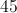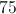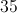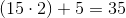# High School Math : How to find the perimeter of an acute / obtuse isosceles triangle

## Example Questions

### Example Question #1 : How To Find The Perimeter Of An Acute / Obtuse Isosceles Triangle

One side of an acute isosceles triangle is 15 feet.  Another side is 5 feet.  What is the perimeter of the triangle in feet?